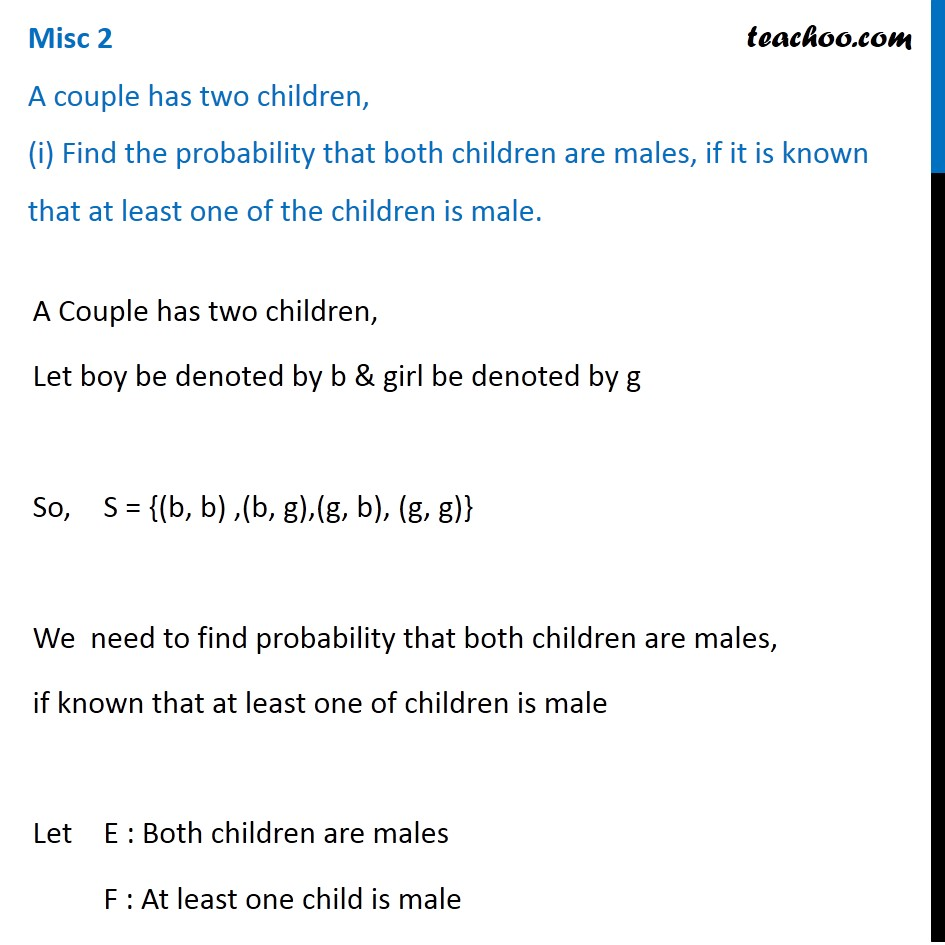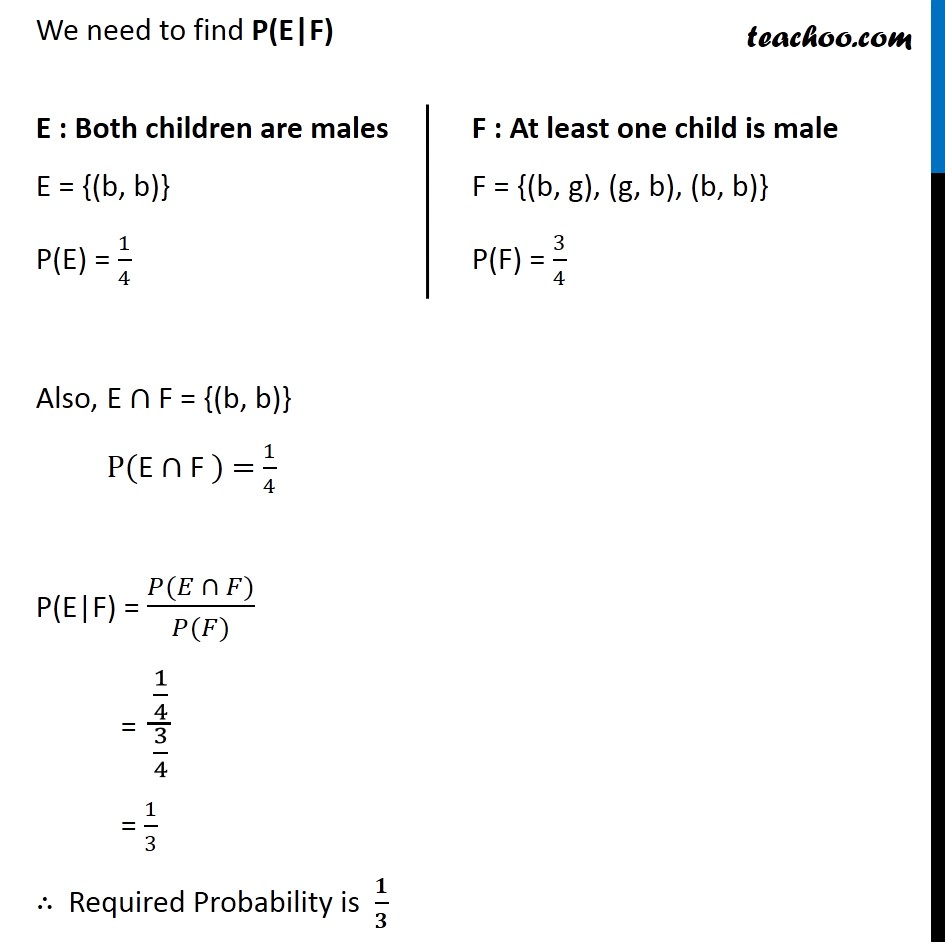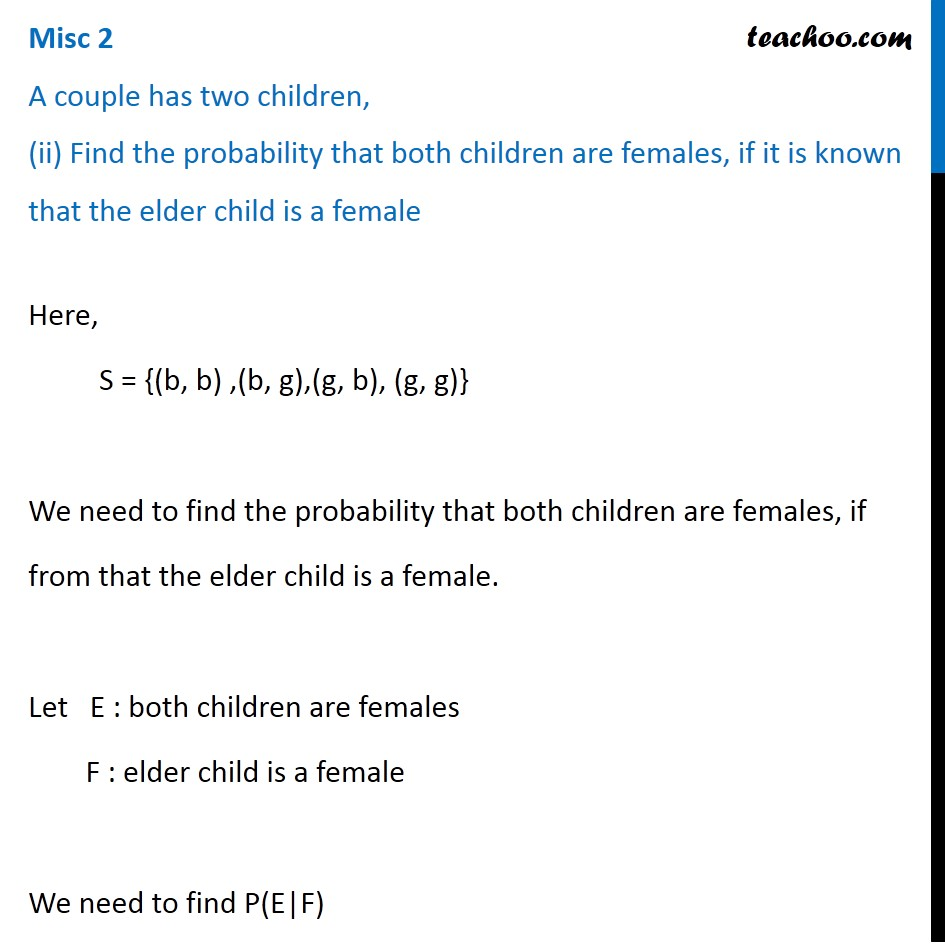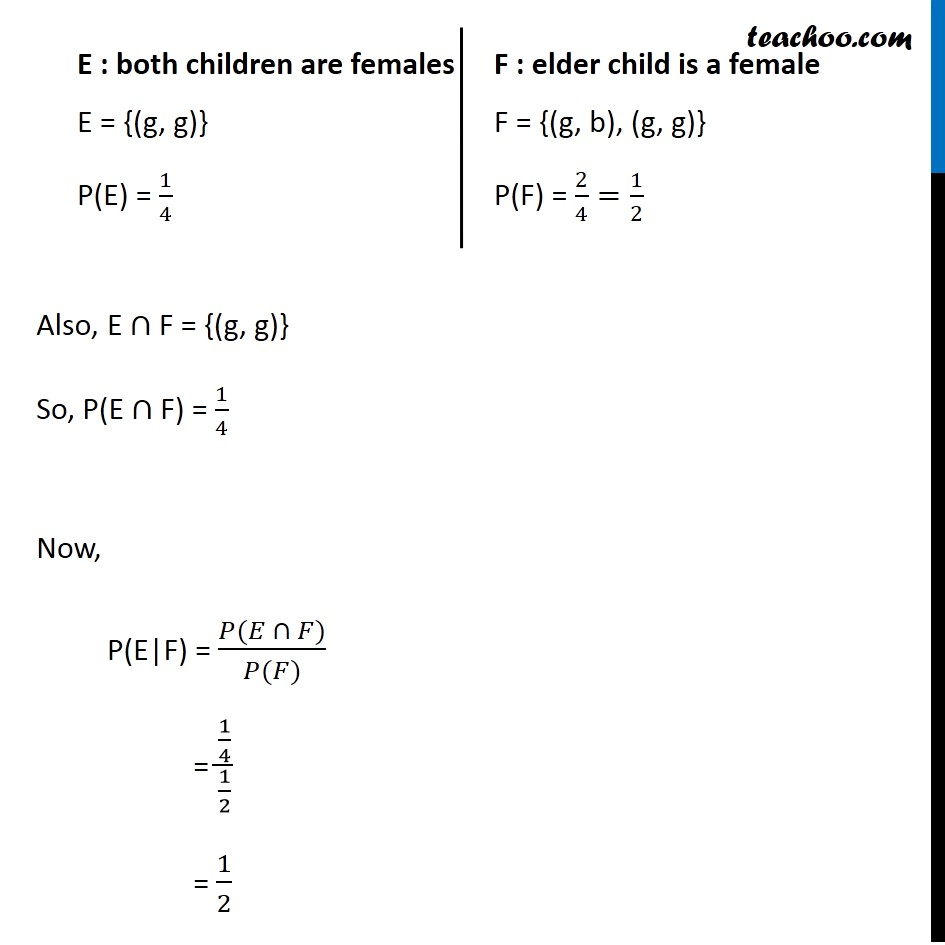Subscribe to our Youtube Channel - https://you.tube/teachoo

1. Chapter 13 Class 12 Probability
2. Serial order wise
3. Miscellaneous

Transcript

Misc 2 A couple has two children, (i) Find the probability that both children are males, if it is known that at least one of the children is male.A Couple has two children, Let boy be denoted by b & girl be denoted by g So, S = {(b, b) ,(b, g),(g, b), (g, g)} We need to find probability that both children are males, if known that at least one of children is male Let E : Both children are males F : At least one child is male We need to find P(E|F) Also, E ∩ F = {(b, b)} P(E ∩ F ) = 1/4 P(E|F) = (𝑃(𝐸 ∩ 𝐹))/(𝑃(𝐹)) = (1/4)/(3/4) = 1/3 ∴ Required Probability is 𝟏/𝟑 E : Both children are males E = {(b, b)} P(E) = 1/4 F : At least one child is male F = {(b, g), (g, b), (b, b)} P(F) = 3/4 Misc 2 A couple has two children, (ii) Find the probability that both children are females, if it is known that the elder child is a femaleHere, S = {(b, b) ,(b, g),(g, b), (g, g)} A We need to find the probability that both children are females, if from that the elder child is a female. Let E : both children are females F : elder child is a female We need to find P(E|F) E : both children are females E = {(g, g)} P(E) = 1/4 F : elder child is a female F = {(g, b), (g, g)} P(F) = 2/4=1/2 Also, E "∩" F = {(g, g)} So, P(E "∩" F) = 1/4 Now, P(E|F) = (𝑃(𝐸 ∩ 𝐹))/(𝑃(𝐹)) = (1/4)/(1/2) = 1/2

Miscellaneous